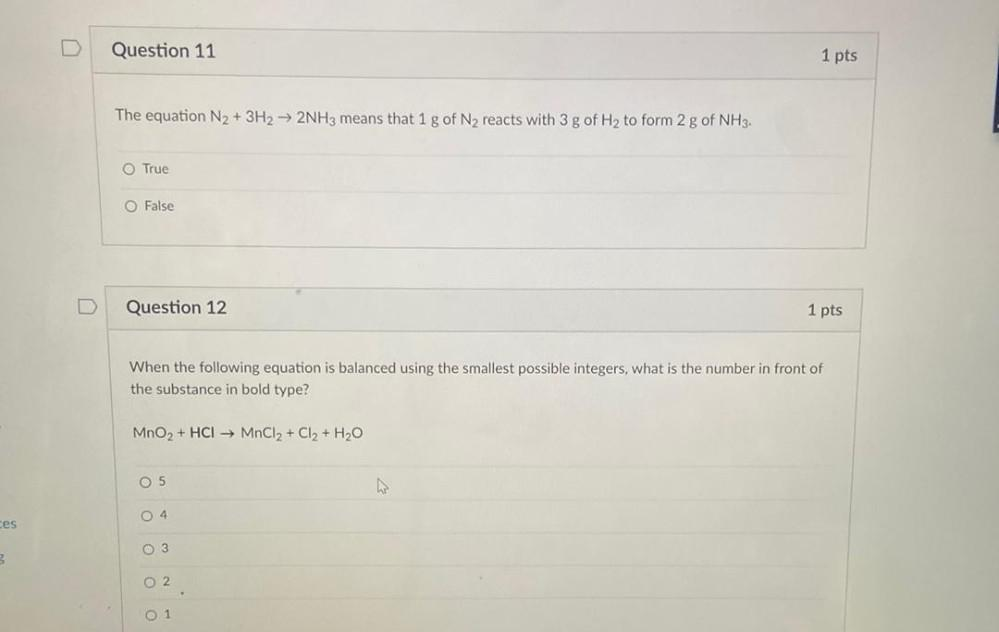Question:

# Question 11 1 pts The equation N2 + 3H2 → 2NH3 means that 1 g of N2 reacts with 3 g of Hy to form 2 g of NH3. O True O False QueQuestion 11 1 pts The equation N2 + 3H2 → 2NH3 means that 1 g of N2 reacts with 3 g of Hy to form 2 g of NH3. O True O False Question 12 1 pts When the following equation is balanced using the smallest possible integers, what is the number in front of the substance in bold type? MnO2 + HCI MnCl2 + Cl2 + H2O O 5 04 ces O 3 O 2 0 1